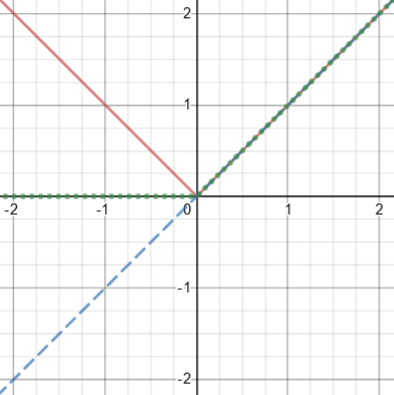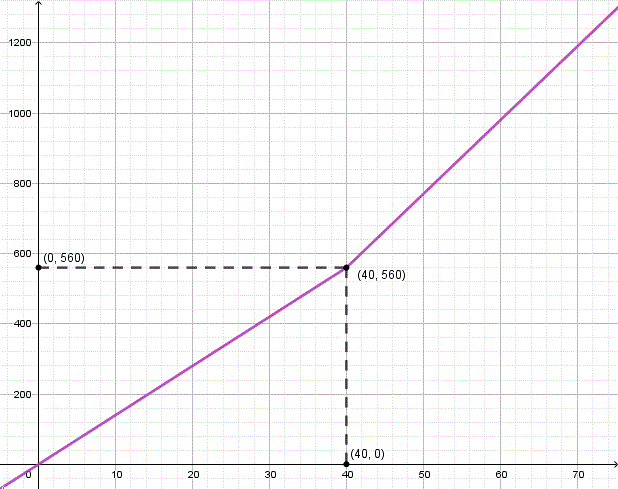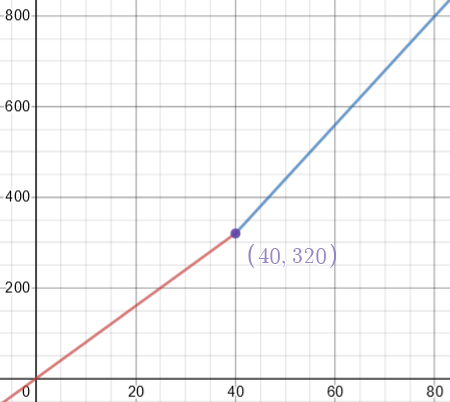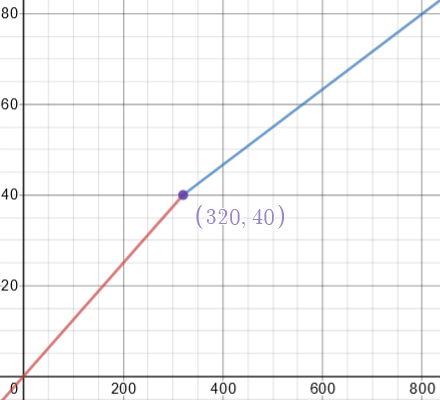# Un-piecing and Inverting a Piecewise Function

#### (A new question of the week)

Though students often think piecewise-defined functions are unnatural, they are actually quite common in real life – after all, the world is not all once piece! In particular, they show up in various financial situations, such as taxes and pay rates. Inverses, likewise, are commonly needed in real life, in order to solve for an appropriate input. What happens when you combine the two concepts? It turns out not to be very difficult (in typical real-life cases); but we don’t see it taught very often. Let’s take a look.

## Pay rates and overtime

This question was sent to us in early February by Brennan:

I am attempting to find a graphable function of this problem here. I know how to calculate each part of this problem individually. However, I am having struggle finding the function of (y) to (x).

How many hours (y) must one work at a job, at varying pay rates (x) to equate to a specific net income value (t)? Any hours above 40 hours will have the pay rate multiplied by 1.5, this is the overtime.

An example would be;

If I want to earn $760 in one week, How many hours would I have to work at$19/hr? 40hours.

How many hours, if I was paid $14/hr? ~49.5hours with 9.5 of those hours at 1.5x pay rate. How many hours, if I was paid$22/hr? ~34.5hours

How many hours, if I was paid $322/hr? ~2.4hours Ignoring the 1.5x overtime pay, the base formulas for calculating the various parts of the equation are as follows. y = t / x x • y = t x = t / y Incorporating the overtime pay, the closest I have been able to come to a proper function is as follows. t = x|40 – y| + 1.5x(y – 40) However, this formula still breaks due to the conditional statements of the overtime pay. Pay with overtime is inherently “piecewise”, as the behavior of the function changes when time exceeds 40 hours. The same sort of thing is true of many income tax schemes, and the like. Let’s check one of his examples (the most interesting one): If he works 49.5 hours at$14 per hour, with overtime, will be really earn $760? In the first 40 hours (regular time), he will earn $$40\times 14 = 560$$. Then he works 9.5 hours at the overtime rate, which is $$1.5\times 14 = 21$$ per hour. This earns him $$9.5\times 21 = 199.50$$. The total is $$560+199.50=759.50$$, which is reasonably close to$760. (We’ll see later whether we can do better with the formula we work out.)

But his formula for the total earned is wrong, so he’s not ready to invert it and find a formula for the hours required. There’s a lot here to explore!

Hi, Brennan.

When you say you are seeking a “graphable function”, I think you mean that you want to write the total income (t) as a function of the hours worked (y), with the pay rate (x) as a parameter. You can do this as a piecewise-defined function, and you have come close to writing it as a single algebraic expression instead, by means of the absolute value function. However, your goal is to invert that function, in order to solve for y as a function of x, and your function is not amenable to inversion by algebraic means.

Many students feel like piecewise functions are “non-mathematical”, and feel compelled to turn them into a single expression; but generally it is better to leave it in piecewise form. On the other hand, the absolute-value form is an interesting idea, so we’ll be exploring that idea as well.

## Making the piecewise function

I am going to change the names of variables so that I can more easily remember what each means:

r = pay rate in dollars per hour (a parameter, fixed for a given situation)

x = hours worked (which will be our independent variable at first)

y = total income in dollars

No doubt you were able to write this in piecewise form, though you didn’t show it — this is fairly straight-forward based on the information given:

y = { rx,                  if x ≤ 40

{ 40r + 1.5r(x - 40),  if x > 40

That last line can be simplified to 1.5rx – 20r.

Brennan’s x (pay rate), y (hours), and t (net income value) have been changed to r (pay rate), x (hours), and y (net income value), in part because t sounds like time, and because r sounds more like a fixed parameter.

The piecewise definition reflects that fact that for up to 40 hours we don’t have an overtime calculation, while for more than 40 hours we do: a rate of r for the first 40 hours and $$1.5r$$ for the remaining $$x-40$$ hours.

## Turning it into a single expression

What you have written as a single expression,

y = r |40 - x| + 1.5r(x - 40)

using my variables, is not correct — for instance, if x = 20, it gives y = 20r + 1.5r(-20) = -10r, and the income should not be negative!

We don’t know how Brennan got his expression; the last term is appropriate when overtime applies, but the absolute value appears to be an attempt to compensate. There are probably several ways this could be done, but this doesn’t quite make it. Taking another test case, if $$x=40$$, we get $$y=0$$, which definitely isn’t good.

But the idea is good; we can use the fact that

(|x| + x)/2 = { 0, x ≤ 0

{ x, x > 0

to rewrite my function above as

y = r(x + (|x - 40| + x - 40)/4)

Here is a graph of $$y=|x|$$, $$y=x$$, and $$y=\frac{|x|-x}{2}$$, to show how that formula works:The variable (blue) and its absolute value (red) are identical on the right, so the average leaves it unchanged; they are opposites on the left, so their average is zero.

What Doctor Rick has done to apply it is to rewrite the piecewise function $$f(x)=\left\{\begin{matrix}rx & \text{if }x\le 40\\ 40r + 1.5r(x – 40) & \text{if }x> 40\end{matrix}\right.$$ as $$f(x)=\left\{\begin{matrix}r(x+0) & \text{if }x\le 40\\ r(x+\frac{x – 40}{2}) & \text{if }x> 40\end{matrix}\right.$$

Then, replacing $$x$$ in the identity with $$x-40$$,  $$\frac{x+|x|}{2}=\left\{\begin{matrix}0 & \text{if }x\le 0\\ x & \text{if }x> 0\end{matrix}\right.$$ becomes $$\frac{(x-40)+|x-40|}{2}=\left\{\begin{matrix}0 & \text{if }x\le 40\\ x-40 & \text{if }x>40\end{matrix}\right.$$

This leads to his equation, $$f(x) = r\left(x + \frac{x – 40 + |x – 40|}{4}\right) = r\left(\frac{5x – 40 + |x – 40|}{4}\right)$$

We can’t easily invert a function like this, to solve for x in terms of y; I’m going to go back to the piecewise-defined function for that. However, you might want to use the same idea later.

As I’ve mentioned, piecewise definitions are usually easier to work with, as we’ll see.

## Graphing the function

Are you familiar with the shape of the graph of this function? I have attached a graph for r = 14. It consists of two straight-line segments.One important feature is that it is one-to-one, so an inverse function is possible; not all piecewise functions are. Another is that point where the slope changes, whose x-coordinate is important in the original function, and whose y-coordinate will be used in the inverse.

## Inverting the piecewise function

Now we want to invert the function, which means to find what x is for a given y. We do that by inverting each piece separately, and determining when that piece is used. (We aren’t going to swap x and y, as we usually do, because they have specific meanings (hours worked and total income, respectively). For graphing, we will have to swap them, because Desmos always makes x horizontal.)

The piecewise-defined function for y as a function of x, above, reflects this by defining two linear functions, one to be used when x < 40 and the other to be used when x > 40. Now, to write a piecewise function for the inverse, we only need to note that we will use the former line when y < 40r (560 in the example) and the latter when y > 40r:

If y ≤ 40r then y = rx

If y > 40r then y = 1.5rx - 20r

Now I solve each of the equations for x:

x = { y/r,             y ≤ 40r

{ (2y/r + 40)/3,   y > 40r

We’ve changed the limits from values of x to the corresponding values of y, and then solved each linear function for x as a function of y.

The original function, in simplified form, was $$f(x)=\left\{\begin{matrix}rx & \text{if }x\le 40\\ 1.5rx – 20r & \text{if }x> 40\end{matrix}\right.$$

The inverse function is $$f^{-1}(y)=\left\{\begin{matrix}\frac{y}{r} & \text{if }y\le 40r\\ \frac{2y+40r}{3r} & \text{if }y> 40r\end{matrix}\right.$$

I’ve graphed both functions for a different example case, $$r=8$$; I can’t graph them on the same axes, as we often do to show the relationship between function and inverse, because they need different scales. The original function is $$f(x)=\left\{\begin{matrix}8x & \text{if }x\le 40\\ 12x – 160 & \text{if }x> 40\end{matrix}\right.$$The inverse is $$f^{-1}(y)=\left\{\begin{matrix}\frac{y}{8} & \text{if }y\le 320\\ \frac{y+160}{12} & \text{if }y> 320\end{matrix}\right.$$That’s as far as I’ll go right now. If you wish, you could use the absolute-value idea to rewrite this as a single expression. However, you may not need it. Let me just check that it gives the correct solution to one of your examples: How many hours would a person have to work to earn $760 at$14/hour? With r = 14, 40r = 560, so 760 is greater than that and we use the second formula:

(2(760)/14 + 40)/3 = 49.524

Recall that Brennan had found (by trial and error?) that he would need about 49.5 hours, which was a little too low; now we have a more accurate answer from our formula.

As happens too often, Brennan never came back to read this answer; perhaps his email address was incorrect. But let’s take it a little further (and maybe he’ll see the answer here).

## Making a single-expression inverse

Although, again, it isn’t really necessary, we’ll turn the inverse function into a single expression.

We rewrite our piecewise inverse function $$f^{-1}(y)=\left\{\begin{matrix}\frac{y}{r} & \text{if }y\le 40r\\ \frac{2y+40r}{3r} & \text{if }y> 40r\end{matrix}\right.$$ as $$f^{-1}(y)=\left\{\begin{matrix}\frac{y}{r}+0 & \text{if }y\le 40r\\ \frac{y}{r}+\frac{-y+40r}{3r} & \text{if }y> 40r\end{matrix}\right.$$ and obtain $$f^{-1}(y)= \frac{y}{r}+\frac{-(y-40r)-|y-40r|}{6r}= \frac{6y-(y-40r)-|y-40r|}{6r}= \frac{5y+40r-|y-40r|}{6r}$$

I have verified that this produces the same graph as before.

This site uses Akismet to reduce spam. Learn how your comment data is processed.